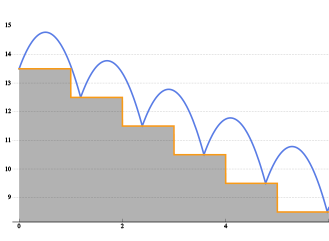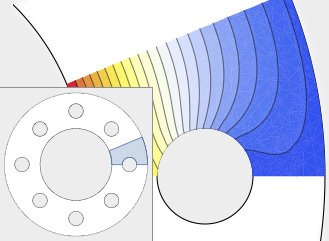# Differential Equation Solving

## Symbolic Delay & Hybrid Differential Equations »

 Mathematica Version 10 adds many significant enhancements to its traditional strength in symbolic calculus. For the first time, symbolic solutions to hybrid differential equations with events and delay differential equations are possible. All discrete calculus operations are extended with newly developed algorithms motivated by probability and statistics. New dedicated commands for curve geometry as well as newly added special functions automate many tasks. Taken together, these developments will lead to smoother workflow for a wide range of applications, starting from college calculus to research level. Learn More## Partial Differential Equations »

 Mathematica Version 10 extends its numerical differential equation-solving capabilities to include the finite element method. Given a PDE, a domain, and boundary conditions, the finite element solution process - including grid and element generation - is fully automated. Stationary and transient solutions to a single PDE or a system of partial differential equations are supported for one, two, and three dimensions. Learn More